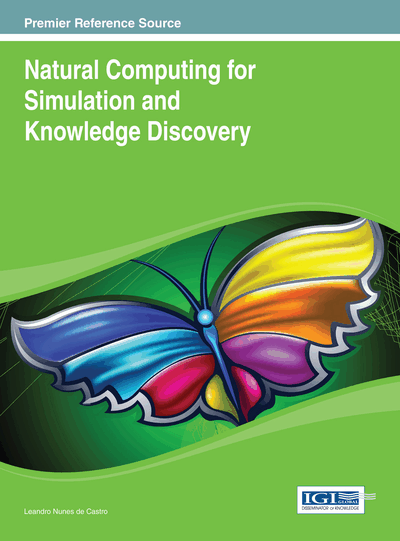# P Colonies of Capacity One and Modularity

Luděk Cienciala (Silesian University in Opava, Czech Republic), Lucie Ciencialová (Silesian University in Opava, Czech Republic) and Miroslav Langer (Silesian University in Opava, Czech Republic)
DOI: 10.4018/978-1-4666-4253-9.ch007
Available
\$37.50
No Current Special Offers

## Abstract

In this paper, the authors continue the investigation of P colonies introduced in Kelemen, Kelemenová, and Paun (2004). This paper examines a class of abstract computing devices composed of independent agents, acting and evolving in a shared environment. The first part is devoted to the P colonies of the capacity one. The authors present improved results concerning the computational power of the P colonies with capacity one and without using checking programs. The second part of the paper examines the modularity of the P colonies. The authors then divide the agents into modules.
Chapter Preview
Top

## 2. Definitions

Throughout the paper we assume that the reader is familiar with the basics of the formal language theory.

We use NRE to denote the family of the recursively enumerable sets of natural numbers. Let Σ be the alphabet. Let Σ* be the set of all words over Σ (including the empty word ε). We denote the length of the word w∈Σ* by |w| and the number of occurrences of the symbol a∈Σ in w by |w|a.

A multiset of objects M is a pair M = (V, f), where V is an arbitrary (not necessarily finite) set of objects and f is a mapping f: V → N; f assigns to each object in V its multiplicity in M. The set of all multisets with the set of objects V is denoted by V*. The set UV is called the support of M and is denoted by supp(M) if for all

xU f(x)≠0 holds. The cardinality of M, denoted by |M|, is defined by |M| = Σa∈V f(a). Each multiset of objects M with the set of objects U = {a1, …, an} can be represented as a string w over alphabet U, where

Obviously, all words obtained from w by permuting the letters represent the same multiset M. The ε represents the empty multiset.

## Complete Chapter List

Search this Book:
Reset